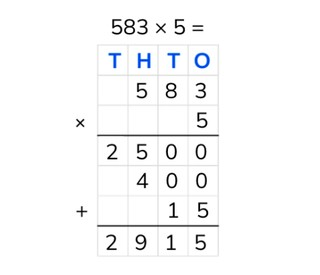Partial products algorithm with numbers to 1,000

# Partial products algorithm with numbers to 1,000

Students learn to multiply partial products with numbers to 1,000.8,000 schools use Gynzy92,000 teachers use Gynzy1,600,000 students use Gynzy

## General

Students learn partial products multiplication with a number to 1,000.

## Standards

CCSS.Math.Content.4.NBT.B.5

## Learning objective

Students will be able to multiply partial products with a number less than 10 and a number to 1,000.

## Introduction

Spin the wheel for the first number and drag a cover away for the second number. Fill in the numbers on the orange and blue lines. Then the students solve this problem.

## Instruction

Explain that in partial products multiplication you put the numbers one on top of the other. Then you multiply them together by calculating from left to right. Explain that you put the larger number on top and the other number underneath. With partial products you work from left to right. So you start by multiplying the hundreds numbers. You multiply these together. Then you multiply then tens and then the ones. You put all of these answers into the chart and write down the intermediate problems. If you add all the numbers in the chart together, you have the final answer. You put this answer at the bottom of the chart. Next there is a problem in a chart, each one of which with a different row marked in yellow. Ask the students if they know which intermediate problem belongs with each colored row. This is how you check if the students know what steps they need to take. After this you explain that if you have a multiplication problem with 0 you don't have to write down the answer. When you multiply a number by 0, the answer is always 0. Next you show a chart for which the intermediate steps need to be filled in. Have the students write these numbers down and hold them up, so that you can check their answers. Then practice partial products with two problems, the first one of which has the chart filled in and the other chart still must be filled in.

Check whether the students can multiply partial products by asking the following questions:
- Why is it useful to be able to calculate partial products?
- What do the letters T H T O mean?
- Where do you always start when calculating partial products?
- Solve this problem using partial products: 330 × 6

## Quiz

The students test their understanding of partial products multiplication with numbers to 1,000 through ten exercises. For some the students must give the answer, and for others they must fill in the intermediate steps. Some of the THTO-charts have been filled in, and for other exercises the students must fill in the chart themselves.

## Closing

You discuss once again with the students that it is important to be able to multiply using partial products with a number to 1,000, because that is how you can solve problems in steps. Check if the students know that with partial products they must work from left to right. Have the students come up with problems on their own by dragging numbers into the THTO-chart.

## Teaching tips

When students have difficulty with partial products multiplication, you can repeat the meaning of T H T O and how you put the numbers in a THTO-chart. Write out the intermediate problems. You may also label the hundreds, tens, and ones, so that it is obvious what you are multiplying together.

### The online teaching platform for interactive whiteboards and displays in schools

• Save time building lessons

• Manage the classroom more efficiently

• Increase student engagement